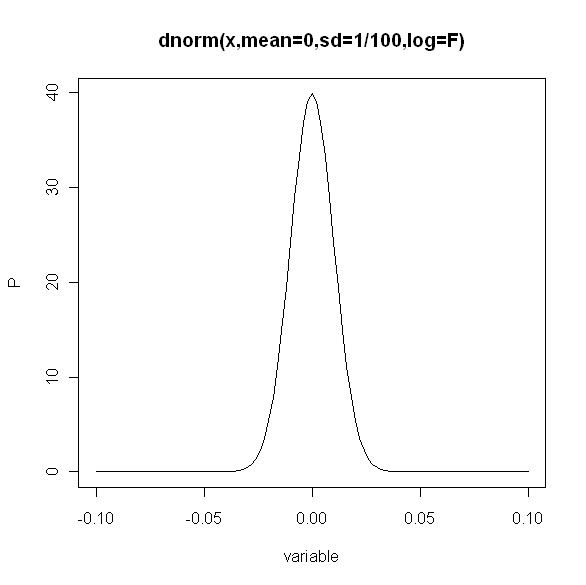# [FAQ] Why am I getting negative A.I.C. scores?

#### TheEcologist

##### Global Moderator
Why am I getting negative A.I.C. scores?

It is correct that negative A.I.C. sent up red flags for you as it may tell you that something went wrong in your analysis - as logically log-likelihoods (or AICs) cant really be negative, well at least, not theoretically or 'technically speaking'. In practice, however, it can actually happen. There are cases in which a given software program will return negative values for something called the "negative log-likelihood density" function.
Probably one of the most likely ways this can arise is through the use of very narrow continuous probability density functions. People often use density functions (e.g. the normal distribution; the function dnorm() in R) to calculate (log) likelihood, when these get very narrow (as below) ”problems“ may arise.As you can see the density in this very narrow normal pdf can go up to pretty high values [here approx 39.89423, the density of x is defined above by dnorm(0,μ=0,σ=1/100)]. It is no surprise then that this will lead to positive log likelihoods - as the density goes above 1, the log-likelihood will be positive, and as a result the calculated AIC will be negative.

Example: We we calculate the log likelihood (LL) with the normal density function dnorm(μ=0,σ=1/100) for the values in a vector X were X = (-0.03, -0.01, 0, 0.01, 0.03) - which is the sum of the log likelihood of each value of X. You wil find it sums up to a positive value.

$$LL(X) = \sum_{i = 0}^n ln(dnorm(X_{i},{\mu}=100, \sigma = 1/100))$$

#In R
Code:
sum(dnorm(c(-0.03, -0.01, 0, 0.01, 0.03),0,1/100,log=T))
8.431158
Simple calculations then reveal that any AIC value calculated will be negative (AIC < 0):

AIC = -2 LL + 2k

This will happen any time a likelihood curve, like in the graph, is narrow.

Oke, so what do you do now? Throw out your analysis? Start over? Get other software?
Don't worry, there is no need to panic as it turns out that the negative value of the negative log-likelihood does not matter in practice [note: unless you are working with a discrete likelihood function]. In model selection with AIC the thing that truly matters is differences between the negative AIC scores. Just look for the model with the smallest AIC.

If you're still having trouble with this topic feel free to start a thread on the forum, and be sure to check out our guidelines for efficient posting.